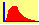Limiting Behavior of the Binomial Tree Module

Introduction to the Problem

In the binomial option pricing model you may think it is highly artificial to assume that underlying stock price can only go up or down at the end of the period.  For example, applied to a 3-month IBM option it is clearly unrealistic to assume that IBM will generate either a positive or negative return three months from now.  However, this assumption is more palatable if we assumed that IBM will generate either a positive or negative return 10-seconds from now.  Alternatively, you may say well really there are three possibilities here, up, down or no change.  This would imply working with a trinomial distribution as opposed to the binomial case.  Although we could work with either assumption, in this example we will choose the simpler of the two, the binomial case, to observe how far this can take us. Later we will benchmark it against both the Black-Scholes option pricing model and the trinomial numerical estimation method.

So lets get back to our 10-seconds sub partitioning example.  Ten seconds is a small sub interval of a 3-months time period, but nevertheless it is indeed a mathematically well defined small sub interval of our 3-months period of time.  Hence, consider partitioning the life of an option into smaller and smaller sub intervals of time.  Observe that, the more we partition into smaller sub-periods of time, the greater the number of possible end of maturity prices exist for the underlying asset.  In addition, not all these prices are equally likely.  The most unlikely are the two price paths that assume either all up-ticks are realized in each subinterval of time or vice versa.  Thus the observed frequency distribution for the underlying asset price is not a uniform distribution.  This module, however, will let you explore the behavior of resulting observed frequency distribution.

To see this and other important questions complete the following steps.

Step 1:  In the module to the left, let us examine how the distribution of end of maturity prices for the underlying asset looks under the assumption of increasing the number of partitions within a fixed time period.  First, select the density option.  You can plot the density that results from 10 to 200 partitions of time.  You will quickly observe that the return distribution gets closer and closer to a normal distribution.  If interested, you can read why in the following description of one of the most important theorems in applied statistics, the central limit theorem.  Otherwise skip to step 2.

Central Limit Theorem

Consider a set of independent and identically distributed random variables (xI) each with mean m and variance s2. Suppose there are n random variables and we construct the following statistic (i.e., number).  Sum the n random variables, subtracting n times the mean m from the sum and scale by the standard deviation (i.e., volatility), s, times the square root of n. This is commonly referred to as a Z-score. The central limit theorem proves that the limiting distribution of this statistic, as n gets large, converges upon the standard normal distribution.

You can see the effects of this theorem by conducting an experiment with the module ranging from 10 partitions to 200 partitions.  That is, much of the power of the central limit theorem lies in the fact that in practice it does not require an infinite number of partitions to work.

So how does this help to price options using numerical methods?

Step 2:  Click on the button Price Approximations.  The objective of this subject is to observe how the binomial option price responds when we partition the life of the option into smaller and smaller sub partitions.

As a benchmark we contrast this behavior to the Black-Scholes pricing model and the trinomial pricing model.

Question: What happens to the arbitrage free value of an option in a repeated simple up-/down-tick (i.e., binomial) world when the period of time becomes smaller and smaller?

The answer to question reveals the power of the binomial option pricing model.  By applying this module you will see that in the limit the standard binomial model converges upon the Black-Scholes option pricing model.   Furthermore, use the default data to see how quickly the binomial option pricing converges to the BlackScholes option pricing model.

Consider the default data set.  The Black-Scholes put option price is 2.729812 and the Binomial with 10 partitions (i.e, 10 sub-periods) is 2.604967.   Next, repeatedly click on the >> button under Number of Steps to increase the number of steps by 10 each time.   Observe that the binomial approximation starts converging from above to the Black-Scholes put price.  An extremely close approximation is obtained after even 300 steps:

Next, set the number of steps back to 9 (i.e., not 10).  This lets you observe the path to convergence when starting with an odd number of steps.  Again, repeatedly click on the >> button under Number of Steps:

The path to convergence for this at-the-money option depends upon using an odd or even number of steps.   With an even number of steps, convergence is from above while for an odd number of steps, convergence is from below.  As a result, quicker convergence is obtained by taking the average of n and n+1 steps.

In conclusion, this reveals that this simple model can be extremely useful for providing a method for computing a numerical solution to various option pricing problems.   To reinforce the important principles you should now work through the following exercise designed for the FTS Binomial Limit Module.